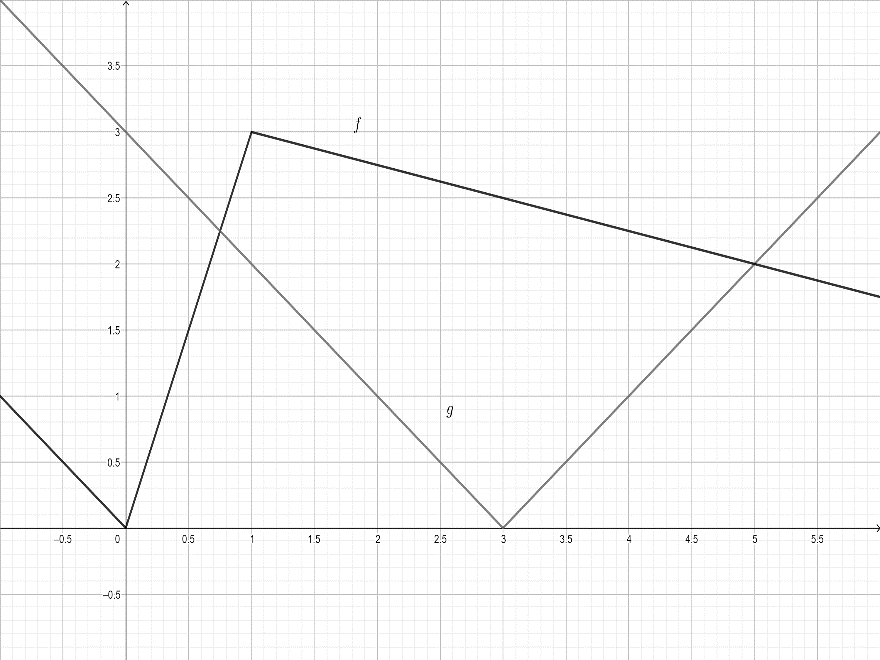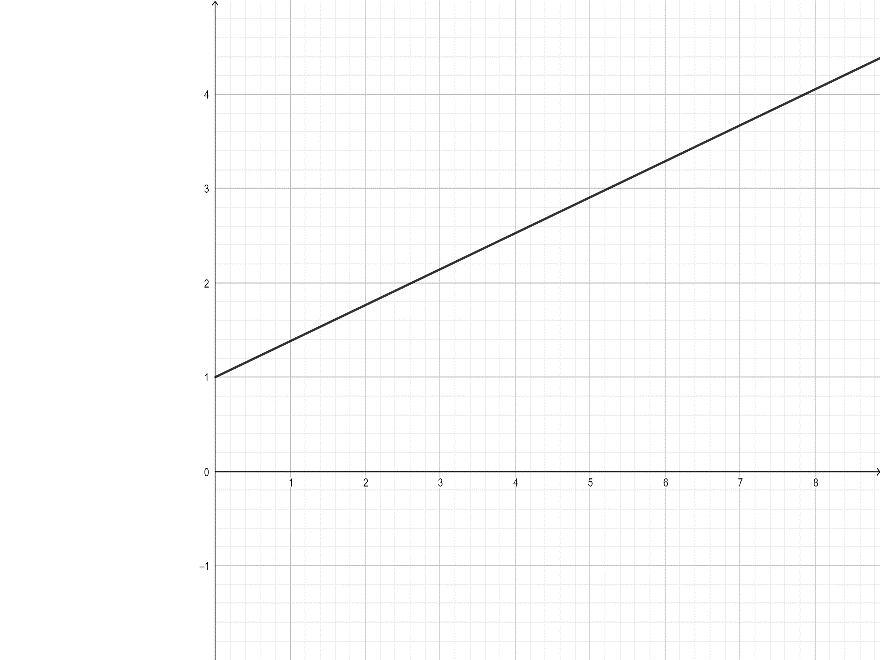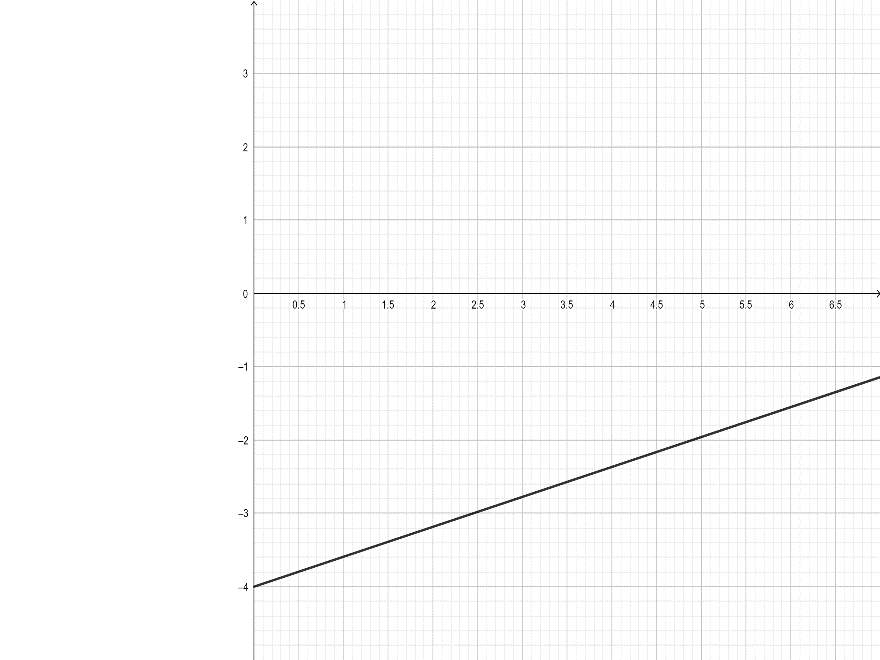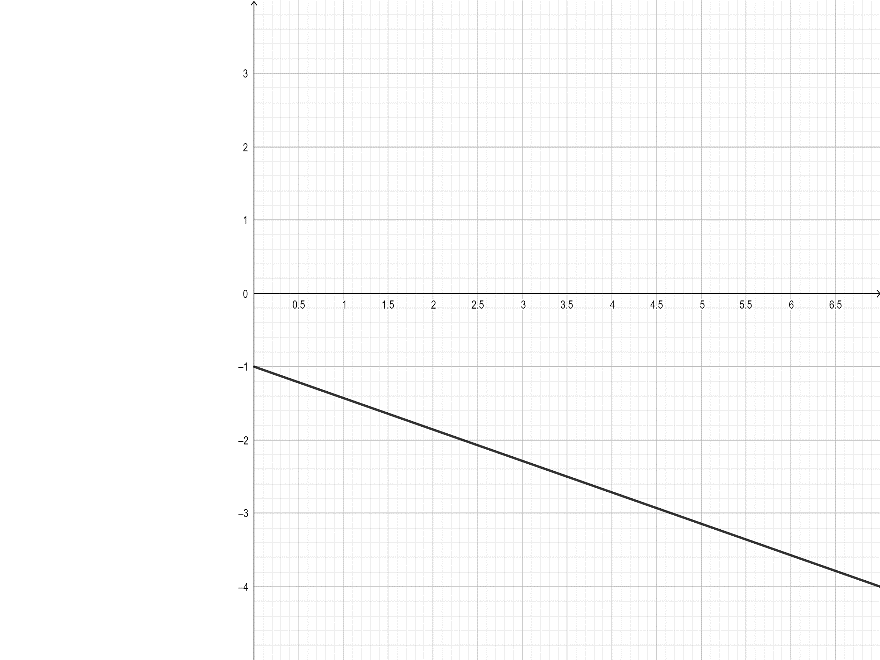Want to see math figures in pictures? Learn about the various types of graphs from TutoryEye.

# Learn all the What, Why, & How about Different Types of Graphs

Mathematical data with two or more variables can be expressed diagrammatically through the use of graphs. Find out more about them with Tutoreye.## Some of our best Graphs Tutors

7 Tutors available

## Top things to learn under Graphs from the online tutor

“Graphs” is an easy and scoring section in the exams, and something that you can use a good deal in your future PowerPoint Presentations:

• What is a Graph?
• Bar Graph
• Line Graph
• Histogram
• Pie Chart
• Venn Diagram
• Box & Whisker Chart
• Pictographs
• Exponential Graphs
• Logarithmic Graphs
• Trigonometric Graphs
• Frequency Distribution Graphs

Question 1: If f and g are the functions whose graphs are shown, let ux=f(x)g(x) and v(x) = f(x)/g(x)• Find u'(1)
• Find v'(5)

Explanation: To find the derivative of the function u(x) and v(x), we can use the product rule and the quotient rule of the derivative respectively.

• Find u'(1)

So, if ux=f(x)g(x).

Then, we know that u'x=f'xgx+g'xf(x)

After analyzing the graph, we can see that: f(1)=3, f'(1)=3. And, g(1)=2, g'(1) = -1.

So, u'(1)=f'(1)g(1)+g'(1)f(1)

Let us plug the values.

u'1=3×2+-1×3=6-3 = 3

So, u'1=3

• Find v'(5)

We have given v(x) = f(x)/g(x).

Then, using the quotient rule,

v'x=gxf'x-fxg'x/(g(x))^2

Looking at the graph, we have: f(5)=2, f'(5)=-1/4. And, g(5)=2, g'(5)=1.

Now, let us plug these values.

v'5=g(5)f'(5)-f(5)g'(5)/g(5)^2

v'5=2×(-1/4)-2×1/(1)^2= (-1/2)-2/1=-5/2

Hence, v'5=-5/2

Question 2: Which graphs represent an object moving in the positive direction?

An object moves in the positive direction if its velocity is positive. The direction of motion cannot be stated by analyzing the slope of the velocity-time graph.

Example:Question 3: Which graphs represent an object moving in the negative direction?

The direction of an object would be negative if it has a negative velocity. The direction of motion cannot be defined by the slope of the velocity-time graph.

The positive or negative slope of the velocity-time graph does not state the positive or negative direction. It just describes how velocity is changing with respect to time.

Example:Math Tutoring TopicsGet started with a demo session to resolve your queries!Live Graphs Tutoring At Lowest Fee - \$7.49 For 30 Mins/MonthChat With Graphs Tutors Anytime, Anywhere.`Only Pay For Time When You Spend In Graphs ClassroomRefill Anytime With Multiple Of \$7.49 For Online Graphs Lessons

Find A Graphs Tutor Online To Scale Up Your Grades With The Help Of One To One Sessions Online at TutorEye.## Are you looking for fast homework help or regular online Graphs tutoring?Our service is fast, convenient and always offered at any given time!

## Don't Go Far, Look Here!We have the most knowledgeable and experienced online Graphs tutors, who are always available to help you solve a tricky problem, complete a challenging Graphs homework assignment or provide useful test preparation tips.We are arguably the best online tutoring website that offers quality tutoring help at a very attractive costOur service is designed to help both high school and college level students.## Some of Our Key Features!

•## Tutor Verification

TutorEye does a thorough back-check before we employ any Graphs tutor online.

•Out tutors holds teaching license, B.Sc, master's, or Ph.D.

•We offer advanced technological virtual classrooms with complete audio, video and even a whiteboard.

•## No-Technical skill needed

You need no special technical skills to use it.

•## Money back guarantee

Money back guarantee if you are not pleased with us.

•## Works for every student

Our online Graphs tutoring is for everyone who wishes to learn Graphs they can be high school or college level students or anyone with interest in Graphs.

## What students say about us?

98% of our students love us…## We Are Different from Others!